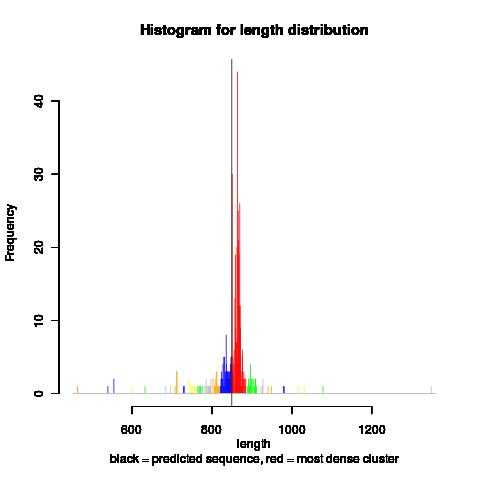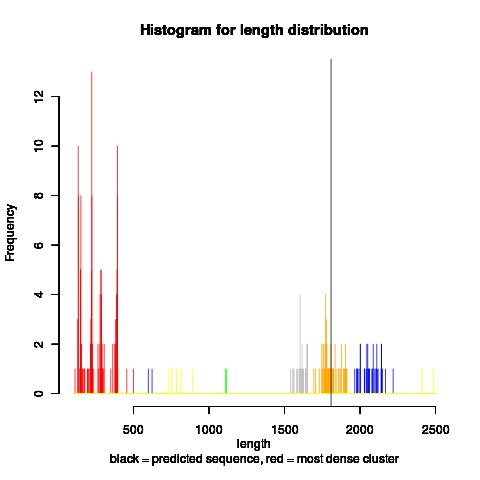# Non-parametric test

##### New Member
Hello everyone,

Context:
I'm am working on a tool that validates predicted genes. At the moment I want to validate the length of the predicted genes with the following approach: for a certain predicted gene I search for similar genes (similar above a certain threshold) in public databases (I use BLAST). I am interested only in the lengths of the genes found by BLAST. The distribution of the lengths of these genes is nonparametric. Here are two examples of length distributions:

1) Predicted length (vertical black line) is among the majority of lengths2) Predicted length is NOT among the majority of lengthsQuestion:
Given a non parametric distribution of values (representing gene lengths):
1) how can I infer if a new value (length of the predicted gene) is part of the distribution?
2)Also, I am looking for a way to quantify how much the new value (my predicted length) is, or is not, among the majority.

What I've tried:
- Intuitively, this is similar to the calculation of the p-value for a parametric t-test.
In my case, I tried (in R) to compute the p-value from :
1) one sample wilcoxon test
2) quantiles normalization of the distribution + one sample t-test

None of the approaches above give the result I need. Is there a standard way of doing this?

Thank you very much for your help!

Monica